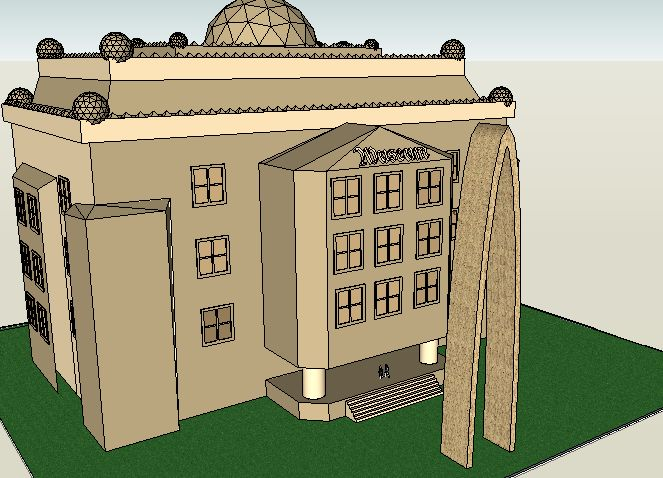SEARCH HOMEMath Central Quandaries & QueriesQuestion from mark, a student: An architect is designing a museum entranceway in the shape of a parabolic arch represented by the equation y = -x2 + 20x, where 0  x  20 and all dimensions are expressed in feet. Determine the maximum height, in feet, of the arch.Hi Mark.The parabola points downwards of course, so its vertex is at the peak. Since you know the equation of the parabola, you can put it into standard form and then just read off the location of the vertex.

For example, if the equation was y = -x2 + 6x, you would want to convert this into the form y - k = A (x - h)2.

Then the vertex is simply (h, k). Here is how I do this:

First I factor out the co-efficient in front of x2:

y = -1( x2 - 6x )

Next, I "complete the square". That means taking half the co-efficient next to the x and squaring this. I both add and subtract it:

y = -1( x2 - 6x + 32 - 32)

Now I can factor the perfect square:

y = -1( (x - 3)2 - 9)

And then move the scalar (the number without the x, that is, the 9) to the other side:

y - 9 = -1 (x - 3)2.

So for this example, the vertex is (3, 9) so the top of the arch would be 9 feet up.

Now you try it with your equation.

Cheers,
Stephen La Rocque.Math Central is supported by the University of Regina and The Pacific Institute for the Mathematical Sciences.